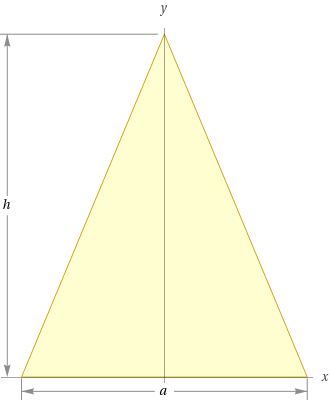Search IntMath
Close

450+ Math Lessons written by Math Professors and Teachers

5 Million+ Students Helped Each Year

1200+ Articles Written by Math Educators and Enthusiasts

Simplifying and Teaching Math for Over 23 Years

# The Isosceles Triangle Theorem in Geometry## What is the Isosceles Triangle Theorem?

The isosceles triangle theorem states that if two sides of a triangle are congruent (or equal in length), then the angles opposite to those two sides are also congruent. This theorem is used to determine the angles in an isosceles triangle, which is a triangle with two sides of equal length.

## How to Prove the Isosceles Triangle Theorem?

The theorem can be proven by using the properties of similar triangles. If two sides of a triangle are congruent, then the angles opposite to those two sides must also be congruent. This is because all three angles in a triangle add up to 180�. Therefore, if two angles in a triangle are the same, then the third angle must be the same as well.

The isosceles triangle theorem can also be proven using the theorem of triangle congruence. According to this theorem, if two sides of a triangle are congruent, then the angles opposite to those two sides are also congruent. Therefore, if two sides of a triangle are equal, then the angles opposite to those two sides must be equal.

## Examples of the Isosceles Triangle Theorem

The theorem can be used to solve for the angles in an isosceles triangle. Consider the following example: An isosceles triangle has two sides of length 6 cm and two angles of measure x and y. Using the isosceles triangle theorem, we can determine that the two angles of the triangle must be congruent. Therefore, x = y.

## Practice Problems

1) In an isosceles triangle, the two sides are 5 cm and 7 cm. What is the measure of the angles opposite to the two sides?
Answer: The angles opposite to the two sides must be congruent. Therefore, the measure of the angles is 45�.

2) In an isosceles triangle, the two angles are 70� and 40�. What is the measure of the sides opposite to the two angles?
Answer: Since the two angles are not equal, the triangle is not an isosceles triangle. Therefore, the measure of the sides cannot be determined.

3) In an isosceles triangle, the two sides are 7 cm and 7 cm. What is the measure of the angles opposite to the two sides?
Answer: The angles opposite to the two sides must be congruent. Therefore, the measure of the angles is 60�.

4) In an isosceles triangle, the two angles are 50� and 50�. What is the measure of the sides opposite to the two angles?
Answer: Since the two angles are equal, the triangle is an isosceles triangle. Therefore, the measure of the sides must be equal. Thus, the measure of the sides is 7 cm.

5) In an isosceles triangle, the two sides are 8 cm and 4 cm. What is the measure of the angles opposite to the two sides?
Answer: The angles opposite to the two sides must be congruent. Therefore, the measure of the angles is 53�.

6) In an isosceles triangle, the two angles are 65� and 75�. What is the measure of the sides opposite to the two angles?
Answer: Since the two angles are not equal, the triangle is not an isosceles triangle. Therefore, the measure of the sides cannot be determined.

## Summary

The isosceles triangle theorem states that if two sides of a triangle are congruent, then the angles opposite to those two sides are also congruent. This theorem can be used to solve for the angles in an isosceles triangle. It can be proven using the properties of similar triangles or the theorem of triangle congruence. Understanding and applying this theorem is an important concept in geometry.

## FAQ

### What is the theorem of an isosceles triangle?

The theorem of an isosceles triangle states that in an isosceles triangle, the angles opposite to the two equal sides are equal.

### What are the properties of an isosceles triangle?

The properties of an isosceles triangle include two sides of equal length, two angles opposite the equal sides of equal measure, and the two unequal sides are referred to as the base and the other side is referred to as the vertex.

## Problem SolverThis tool combines the power of mathematical computation engine that excels at solving mathematical formulas with the power of GPT large language models to parse and generate natural language. This creates math problem solver thats more accurate than ChatGPT, more flexible than a calculator, and faster answers than a human tutor. Learn More.